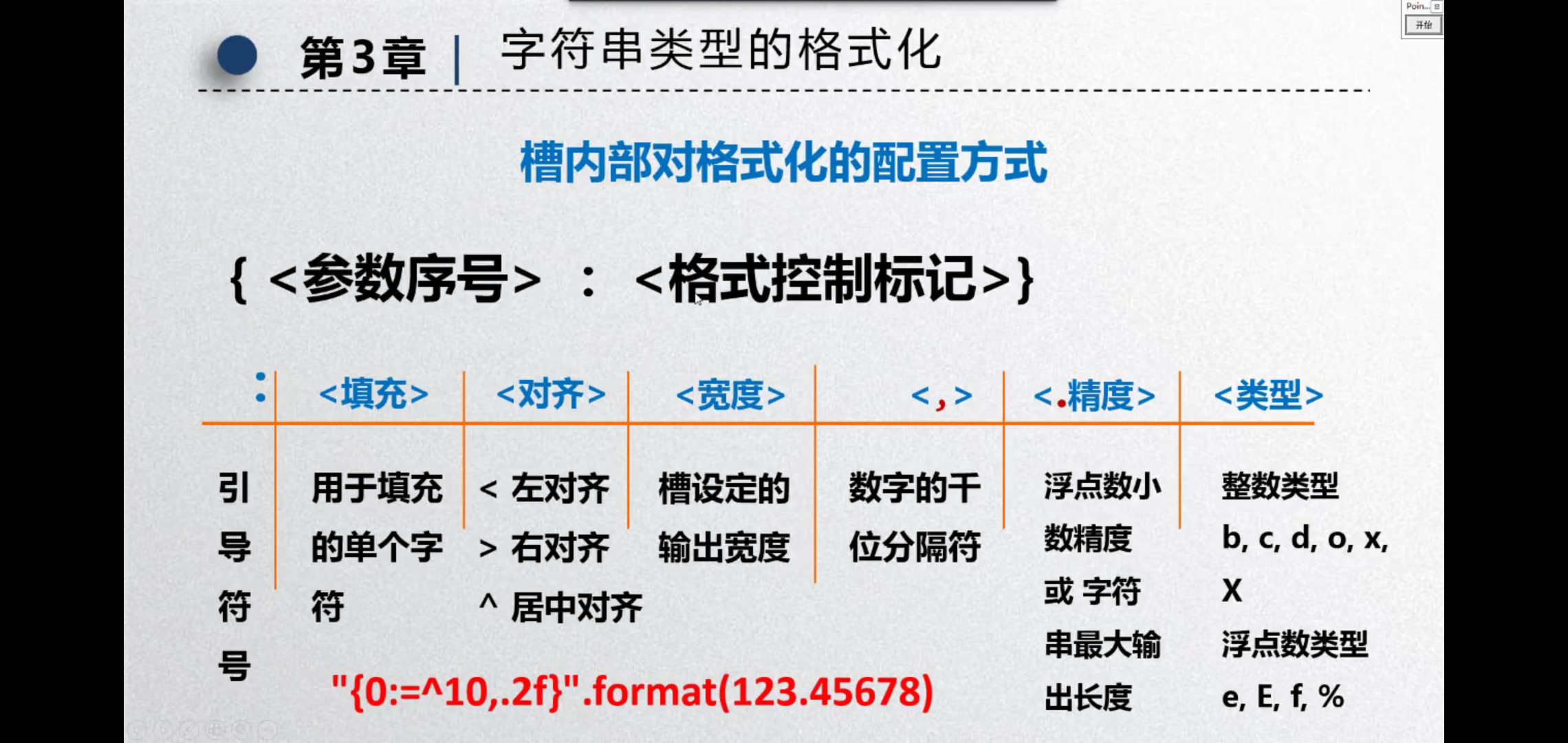• 1.基本数据类型 1.数字类型：整型(int)，浮点型(float)，复数类型(complex) 2.字符串类型：str 3.逻辑类型：bool True False 4.列表类型：list [ ] 有序可修改 5.元组类型：tuple () 有序不可修改 6.集合类型：set {...
基本数据类型1.基本数据类型2.整形中的四种进制4.复数5.数值运算操作6.数值运算函数7.字符串及其相关函数
1.基本数据类型
1.数字类型：整型(int)，浮点型(float)，复数类型(complex)
2.字符串类型：str
3.逻辑类型：bool   True   False
4.列表类型：list [ ]   有序可修改
5.元组类型：tuple ()   有序不可修改
6.集合类型：set  { }  无序不重复
7.字典类型：dict {key：value}   无序
2.整形中的四种进制
1.十进制：1010,99，-217
2.二进制：以0B或者0b开头，0B101
3.八进制：以0O或者0o开头，-0O456
4.十六进制：以0X或者0x开头，0X89
##3.浮点数存在不确定尾数，并不是BUG
0.1+0.2输出0.30000000000004
解决方法：
使用round函数。
round(x,d)：对x四舍五入，d为小数的截取位数。
4.复数
形式：x=2+3j
x.real表示实部，x.imag表示虚部
5.数值运算操作
整除     //
取余     %
幂         **
6.数值运算函数
int()：舍弃小数部分，取整
int(x，base=10)：x是10进制下的10，例如int(“12”,16)=18(16进制下的12等于18)
float()：增加上小数部分一位，变为浮点类型
complex()：增加虚数部分，变为复数类型
max(x1，x2，x3...)：返回最大值
min(x1，x2，x3...)：返回最小值
abs()：返回绝对值

如果对复数a+bj使用abs()函数，结果是复数的绝对值（此复数与它的共轭复数的乘积的平方根），就是 (a2+b2) 开根。

divmod(x，y)：输出商余，(x//y，x%y)
pow(x，y，z)：输出(x**y)%z，z可以省略
7.字符串及其相关函数
字符串和ASC码互相转化的函数
字母—>ASC码：ord()
ASC码—>字母：chr()
多次输出字符串a：a*n
判断子串：x in s
将字符串x逆序输出：y=x[：：-1]
len(x)：返回字符串x的长度
str()：改变数据类型为字符串
hex(x)，oct(x)，bin(x)：整数的十六进制，八进制，二进制的形式字符串
str.lower()，str.upper()：全部转换为小写或者大写
str.islower()，str.isupper()：判断字符串中的所有字符是否为小写，是否为大写
str.isnumeric()：判断是否所有字符为数字
str.isspace()：判断是否所有字符为空格
str.split(sep)：返回一个列表，由str根据sep被分隔的部分组成(字符串—>列表)
str.count(sub)：返回子串sub在str中出现的次数
str.join(iter)：在iter变量的每两个元素之间接一个str，构成一个新的字符串
str.center(width，fillstr)：在字符串str中根据width居中，长度不够左右用fillstr补齐
str.strip(chars)：从str中去掉在其左侧和右侧chars中列出的字符
str.replace(old，new)：new替换掉str中的old部分
str.index(x,i,j)：返回s从i开始到j位置第一次出现x的位置展开全文字符串 列表
• 有序序列：列表、元组、字符串 无序序列：字典、集合 可变序列：列表、字典、集合 不可变序列：字符串、元组
有序序列有：列表、元组、字符串
无序序列有：字典、集合
可变序列有：列表、字典、集合
不可变序列有：字符串、元组


展开全文• 文章目录元组1，元组的定义及其格式创建一个元组打印并查看数据类型2，元组的操作1，访问元组有哪些方法2，怎样重复合并元组3，怎样访问多维元组 1，元组的定义及其格式 元组是一个不可修改的,元素以逗号分隔的以小...
在这里插入代码片# 元组
文章目录1，元组的定义及其格式创建一个元组打印并查看数据类型2，元组的操作1，访问元组有哪些方法2，怎样重复合并元组3，怎样访问多维元组3，如何遍历元祖小结
1，元组的定义及其格式
元组是一个不可修改的,元素以逗号分隔的以小括号包围的有序序列，
可以将元组看成一个不可修改的列表。
格式:
变量名=(元素1，元素2，…)
创建一个元组打印并查看数据类型
a = ('小啦','小小','小娜','小闻')
print(a)   #('小啦','小小','小娜','小闻')
print(type(a))   #<class 'tuple'>

2，元组的操作
1，访问元组有哪些方法
1，怎样通过索引来获取
a = ('小啦','小小','小娜','小闻')
a=a
print(a)   #小娜

,2，怎样遍历元组
使用for循环遍历3，怎样获取子元组
a = ('小啦','小小','小娜','小闻')
a1=a[2::-1]
print(a1)   #('小娜', '小小', '小啦')


2，怎样重复合并元组
怎样合并
a = (1,2)
b = (3,4)
c = a + b
print(c)   #(1, 2, 3, 4)

怎样重复
a = ('666',)
b = a * 4
print(b)   #('666', '666', '666', '666')


3，怎样访问多维元组
mulit_tup = (('王一','王二'),('李一','李二'),('孙一','孙二'))
name = mulit_tup
print(name)   #孙一

3，如何遍历元祖
怎样简洁遍历出一维元组
for name in ('王一','王二','王三'):
print(name)
输出结果：
#王一
#王二
#王三

怎样简洁遍历出二维数组
user_info = (('王一',10),('王二',11),('王三',12))
for user in user_info:
print(user)
输出结果：
#('王一', 10)
#('王二', 11)
#('王三', 12)

小结
***注意：：：***Python中不允许修改元组的数据，包括不能删除其中的元素。
如果元素本身是一个可变数据类型的列表，那么其嵌套项可以被改变。
如果元组中只有一个值，如果没有逗号，变量的类型就是值的类型。
如果有逗号，则表示元组类型。


展开全文• 首先需要知道Python有哪些类型数据元素 数字类型:整型(int),浮点型(float),复数类型(complex) 字符串类型:str 逻辑类型:bool True False 列表类型:list [] 有序 可修改 元组类型:turple () 有序 不可修改 集合类型:...
各类型数据元素相互转化方法及实例一.int整数类型1.int—>float(整形—>浮点数)2.int—>str(整形—>字符串)3.int—>complex(整形—>复数)二.float浮点数类型1.float—>int(浮点数—>整形)2.float—>complex(浮点数—>复数)3.float—>str(浮点数—>字符串)三.complex复数类型一般不转化四.str字符串类型1.str—>int(字符串—>整形)2.str—>float(字符串—>浮点数)3.str—>list(字符串—>列表)五.list列表类型1.list—>str(列表—>字符串)2.list—>turple(列表—>元组)3.list—>dict(列表—>字典)4.list—>set(列表—>集合)(去重)六.turple元组类型1.turple—>str(元组—>字符串)2.turple—>list(元组—>列表)七.set集合类型1.set—>str(集合—>字符串)2.set—>list(集合—>列表)八.dict字典类型1.dict—>list(字典—>列表)2.dict—>str(字典—>字符串)(同上先转列表再转字符串)

数字类型:整型(int),浮点型(float),复数类型(complex)
字符串类型:str
逻辑类型:bool  True  False
列表类型:list []  有序  可修改
元组类型:turple ()  有序  不可修改
集合类型:set {}  无序  不重复
字典类型:dict {key:value}  无序

一.int整数类型
1.int—>float(整形—>浮点数)
方法:float()
a=100
print(type(a))
print(a)
b=float(a)
print(type(b))
print(b)

运行结果:
<class 'int'>
100
<class 'float'>
100.0


2.int—>str(整形—>字符串)
方法:str()
a=100
print(type(a))
b=str(a)
print(type(b))

运行结果:
<class 'int'>
<class 'str'>


3.int—>complex(整形—>复数)
方法:complex()
a=100
print(type(a))
print(a)
b=complex(a)
print(type(b))
print(b)

运行结果:
<class 'int'>
100
<class 'complex'>
(100+0j)


二.float浮点数类型
1.float—>int(浮点数—>整形)
方法:int()
b=float(100)
print(type(b))
print(b)
c=int(b)
print(type(c))
print(c)

运行结果:
<class 'float'>
100.0
<class 'int'>
100


2.float—>complex(浮点数—>复数)
方法:complex()
b=float(100)
print(type(b))
print(b)
c=complex(b)
print(type(c))
print(c)

运行结果:
<class 'float'>
100.0
<class 'complex'>
(100+0j)


3.float—>str(浮点数—>字符串)
方法:str()
b=float(100)
print(type(b))
print(b)
c=str(b)
print(type(c))
print(c)

运行结果:
<class 'float'>
100.0
<class 'str'>
100.0


三.complex复数类型
一般不转化

四.str字符串类型
1.str—>int(字符串—>整形)
方法:int()
a="123"
print(type(a))
print(a)
b=int(a)
print(type(b))
print(b)

运行结果:
<class 'str'>
123
<class 'int'>
123


2.str—>float(字符串—>浮点数)
方法:float()
a="123.456"
print(type(a))
print(a)
b=float(a)
print(type(b))
print(b)

运行结果:
<class 'str'>
123.456
<class 'float'>
123.456


3.str—>list(字符串—>列表)
1.方法:split()(如果想整体变成一个元素，split()内填任何字符串中没有的分割单位都可以)
str1 = 'hello world'
print(type(str1))
print(str1)
str2=str1.split(' ')
#注意split()内不能为空
print(type(str2))
print(str2)

运行结果:
<class 'str'>
hello world
<class 'list'>
['hello', 'world']

2.方法:list()(将字符串str中的每个字符都分割出来成为列表的一个元素)
str1 = 'hello world'
print(type(str1))
print(str1)
str2=list(str1)
print(type(str2))
print(str2)

运行结果:
<class 'str'>
hello world
<class 'list'>
['h', 'e', 'l', 'l', 'o', ' ', 'w', 'o', 'r', 'l', 'd']

五.list列表类型
1.list—>str(列表—>字符串)
1.方法:直接拼接
list1 = ['hello','world']
print(type(list1))
print(list1)
list2 = list1 + list1
print(type(list2))
print(list2)

运行结果:
<class 'list'>
['hello', 'world']
<class 'str'>
helloworld

2.方法:join()
list1 = ['hello','world']
print(type(list1))
print(list1)
# 引号中的内容就是连接各个字符串的字符
list2="".join(list1)
list3=" ".join(list1)
print(list2)
print(list3)
print(type(list2))
print(type(list3))

运行结果:
<class 'list'>
['hello', 'world']
helloworld
hello world
<class 'str'>
<class 'str'>

3.不同类型列表元素转字符串：方法:map()函数 str()函数
lt=["1",2,"a"]
print(type(lt))
print(lt)
ls1=map(str,lt)
ls2="".join(ls1)
print(type(ls2))
print(ls2)

运行结果:
<class 'list'>
['1', 2, 'a']
<class 'str'>
12a


2.list—>turple(列表—>元组)
方法:turple()
list = [1, 2, 3, 4, 5]
print(type(list))
print(list)
tuple = tuple(list)
print(type(tuple))
print(tuple)

运行结果:
<class 'list'>
[1, 2, 3, 4, 5]
<class 'tuple'>
(1, 2, 3, 4, 5)


3.list—>dict(列表—>字典)
方法:zip()
list1 = ['k1','k2','k3']
list2 = [1,2,3]
dt=dict(zip(list1,list2))
print(type(dt))
print(dt)

运行结果:
<class 'dict'>
{'k1': 1, 'k2': 2, 'k3': 3}


4.list—>set(列表—>集合)(去重)
方法:set()
list = [1,2,3,3,3,4]
set1=set(list)
print(type(set1))
print(set1)

运行结果:
<class 'set'>
{1, 2, 3, 4}


六.turple元组类型
1.turple—>str(元组—>字符串)
方法:str()
turple = (1, 2, 3, 4, 5)
print(type(turple))
print(turple)
print(turple)
str = str(turple)
print(type(str))
print(str)
print(str)

运行结果:
<class 'tuple'>
(1, 2, 3, 4, 5)
1
<class 'str'>
(1, 2, 3, 4, 5)
(


2.turple—>list(元组—>列表)
方法:list()
turple = (1, 2, 3, 4, 5)
print(type(turple))
print(turple)
list = list(turple)
print(type(list))
print(list)

运行结果:
<class 'tuple'>
(1, 2, 3, 4, 5)
<class 'list'>
[1, 2, 3, 4, 5]


七.set集合类型
1.set—>str(集合—>字符串)
方法:join()
set = {'a','b','c'}
print(type(set))
print(set)
str=''.join(set)
print(type(str))
print(str)

运行结果:
<class 'set'>
{'b', 'a', 'c'}
<class 'str'>
bac


2.set—>list(集合—>列表)
方法:list()
set = {'a','b','c'}
print(type(set))
print(set)
list=list(set)
print(type(list))
print(list)

运行结果:
<class 'set'>
{'b', 'a', 'c'}
<class 'list'>
['b', 'a', 'c']


八.dict字典类型
1.dict—>list(字典—>列表)
方法:items()
aa={"name":"wy","age":12,"amount":27}
print(type(aa))
print(aa)
bb=[(x,y) for x,y in aa.items()]
print(type(bb))
print(bb)

运行结果:
<class 'dict'>
{'name': 'wy', 'age': 12, 'amount': 27}
<class 'list'>
[('name', 'wy'), ('age', 12), ('amount', 27)]


2.dict—>str(字典—>字符串)(同上先转列表再转字符串)
1.方法:__getitem__()
aa={"name":"wy","age":12,"amount":27}
print(type(aa))
print(aa)
bb=[(x,y) for x,y in aa.items()]
print(type(bb))
print(bb)
print(type(bb.__getitem__(0)))
print(bb.__getitem__(0))
print(bb.__getitem__(1))

运行结果:
<class 'dict'>
{'name': 'wy', 'age': 12, 'amount': 27}
<class 'list'>
[('name', 'wy'), ('age', 12), ('amount', 27)]
<class 'str'>
name
wy


2.方法:json.dumps()
import json
//需要导入json库
dict_1 = {'name':'linux','age':18}
dict_string = json.dumps(dict_1)
print(type(dict_string))
print(dict_string)
print(dict_string)
print(dict_string)

运行结果:
<class 'str'>
{"name": "linux", "age": 18}
{
:


2.方法:str()(强制转换)
dict_2 = {'name':'linux','age':18}
dict_string = str(dict_2)
print(type(dict_string))
print(dict_string)
print(dict_string)
print(dict_string)

运行结果:
<class 'str'>
{'name': 'linux', 'age': 18}
{
:



展开全文• 二、Python中的内置数据结构有哪些？ 列表、元组、字典、集合 三、关于列表的定义？ 列表是用来存储一组有序元素的数据结构 四、列表的存储数据的形式？ 定义列表需要使用方括号（[ ]），列表中的元素都包含在方括号...
• list和tuple都是一个可以放置任意数据类型有序集合，都是既可以存放数字、字符串、对象等 list和tuple都支持负索引 In : nums[-2] Out: 'ad' In : tp[-2] Out: '33'list和tuple都...
• 列表是Python中特有的一种线性数据结构，列表是可变的，有序的，我们可以用选择操作符来改变任意位置的值，和数组不同的是，列表可以同时保存不同类型的元素（异构）。 在CPython中，list是一个存储指针的长度可变...列表
• 数字类型有哪些？ 整型和浮点型 有哪些运算符？ 平时用的加减乘除、整除、模运算、幂运算，比较运算等 他们的属性？ 数字类型没有有序，无序一说。换言之，不存在索引这个概念 数字类型属于不可变数据类型。换言之，...
• list和tuple都是一个可以放置任意数据类型有序集合，都是既可以存放数字、字符串、对象等 list和tuple都支持负索引 In : nums[-2] Out: 'ad' In : tp[-2] Out: '33' list和tuple都支持切片操作 In ...软件测试 自动化测试
• 1、Python基本数据结构有哪些 2、Python数据类型间的互转，举例子 3、数组的遍历和切片 4、有序字典 5、迭代器，优缺点 6、__init__和__new__的区别 7、面向对象的特性、Python中如何体现面向对象 8、pass...
• 序列，存储一些大量的数据，容器是一种数据类型 Python中，可包含其他对象的对象，称之为“容器”。容器是一种数据结构。 常用的容器主要划分为两种：序列（如：列表、元祖等）和映射（如：字典）。序列中，每个元素...列表
• 二、Python中的容器有哪些 - 列表（list） - 集合（set） - 元组（tuple） - 字典（dict） 三、列表（list） list： 有序的数列 在程序中，线性表：数组、链表、栈、队列 python的list底层就是基于双向...
• 4.redis支持的数据类型有哪些?字符串 哈希 列表 集合 有序集合 5.redis的特点和优势 6.运行redis服务端的命令是什么? 7.运行redis客户端的命令是什么? 8.守护进程 9.常用的端口有哪些? 10.复杂度(时间复杂度和空间...
• Python基础中重要的数据类型有元组、列表、字典、字符串和集合，将从以下几个方面进行比较和总结： 1、是否支持边界检查 2、索引方法和特点 3、哪些支持迭代协议 4、元素的访问特点，是顺序的还是无序的 5、...
• 测试基础 你们公司的测试流程是什么 测试方法,黑盒测试方法，白盒测试方法 测试工具用过哪些 测试文档怎么管理 Bug的状态有哪些 bug怎么关闭 什么是回归测试 设计好测试用例的关键是 ...数据类型有哪些，哪些是...软件测试
• 哪些数据类型有序的。python有：字符串、列表、元组、集合、字典、布尔 六种类型 。 其中字典是无序的。集合中字典就是无序集合。3.列表和字典的pop方法什么区别。 列表pop不指定参数就是...
• 3.1.6 C中变量的存储类型有哪些？ 3.1.7 动态规划的本质 3.1.8 实践中如何优化MySQL? 3.1.9 什么情况下设置了索引但无法使用? 3.2.0 SQL语句的优化 3.2.1 数据库索引的底层实现原理和优化 3.2.2 HTTP和HTTPS的...
• 面试官：讲讲Redis的五大数据类型？如何使用？（内含完整测试源码） 今天又一名读者斩获蚂蚁金服Offer，就是这么简单！！ 面试官：讲讲七层网络模型与TCP三次握手与四次断开？ 面试官问我：如果让你设计一个高并发的...# python有序数据类型有哪些python 订阅